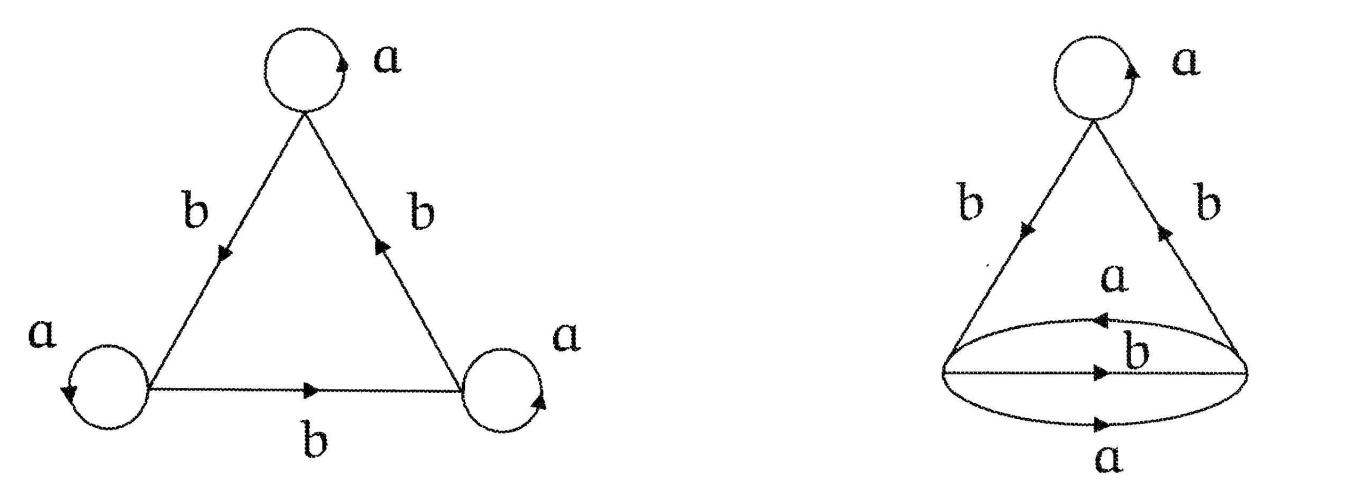# Could I get a few examples of lifts topologically speaking

"Let $p : \tilde{S} \rightarrow S$ be any covering space. By a lift of a closed curve $\alpha$ to $\tilde{S}$ we will always mean the image of a lift $\mathbb{R} \rightarrow \tilde{S}$ of the map $\alpha \circ \pi$ where $\pi : \mathbb{R} \rightarrow S^1$ is the usual covering map."

I was wandering if I could get a few examples of lifts in a visual sense as that is usually the way I can learn things at first. I would be very appreciative. It would help me understand the idea that I read better. Thanks!

Note I am not talking about a path lift.

• But path lifting is the way to understand general lifting. If certain obvious requirements about fundamental groups are satisfied, then a map from a locally path-connected & path-connected space can be lifted to a covering space. The lifting is constructed by explicitly lifting a single point (the basepoint) then using the path (and homotopy) lifting property. See proposition 1.33 in Hatcher. – user40167 Feb 18 '14 at 4:09These give $$3$$-fold covers of $$X$$, which is two circles with a point in common: label those $$a,b$$. Make up a word in $$a,b$$, say $$w=ababa^{-1}b^{-1}$$. Now start at say the left hand vertex of one of the pictured graphs and follow that word through the graph. You are then "lifting" a path in $$X$$ to a path in the covering graph, and should give you a good feel for the process, and how the result differs between the two graphs, or, in the right hand graph, on where you start.